8 December 2019

# Euler bend

### How to use Euler bend interconnects

Euler bends are part of the Interconnect module as of Nazca-0.5.8. Hence, you can draw Euler bends in any xsection. The Euler bend will adjust the number of polygon points to the required mask resolution per layer. The resolution is measured on the central path of the bend. Note that this bend type is also referred to as clothoid, Cornu or Fresnel spiral or bend.

```import nazca as nd
import nazca.demofab as demo

demo.deep.euler(angle=90).put()
nd.export_gds()```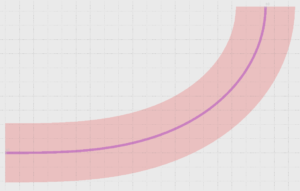You can can combine Euler sections with arc sections in a new cell, as shown in the next code for a 90 degree bend in total.

```# example created by Bright Photonics

import nazca as nd
import nazca.demofab as demo

with nd.Cell(name='Euler1') as euler1:
e1 = demo.deep.euler(angle=30).put()
demo.deep.bend(angle=30).put()
e2 = demo.deep.euler(angle=30).put('b0', flip=True)
nd.Pin('a0', pin=e1.pin['a0']).put()
nd.Pin('b0', pin=e2.pin['a0']).put()

euler1.put()
nd.export_gds()```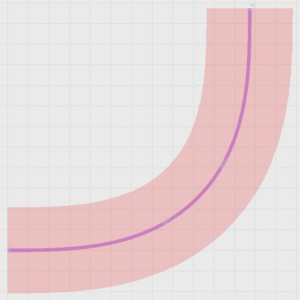The Euler bend has parameters radius and angle and draws the bend from a straight waveguide (infinite curvature) to the end point with the provided angle and radius (curvature = 1/radius) .

```# example created by Bright Photonics

import nazca as nd
import nazca.demofab as demo

with nd.Cell(name='Euler2') as euler2:
angle = 20
nd.Pin('a0', pin=e1.pin['a0']).put()
nd.Pin('b0', pin=e2.pin['a0']).put()

euler2.put()
nd.export_gds()```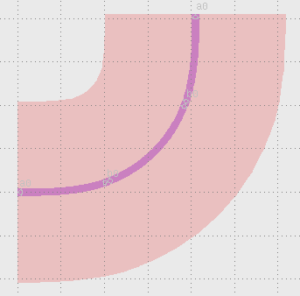## Related Tutorials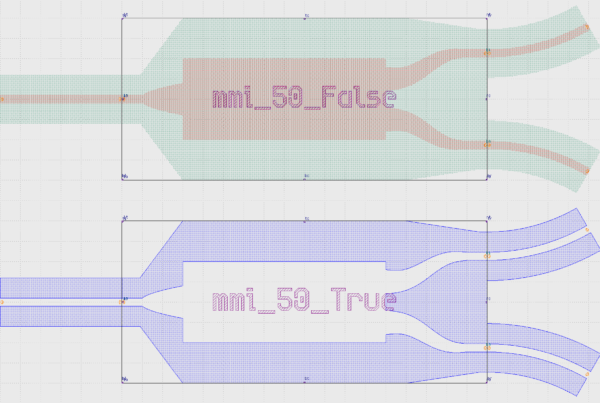In this example we show how to create an inverted MMI with custom interconnects.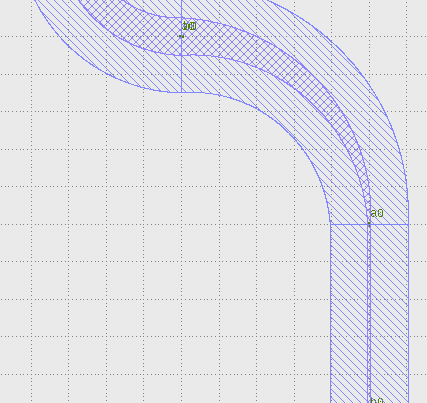In this example we show how to create a free form parametric curve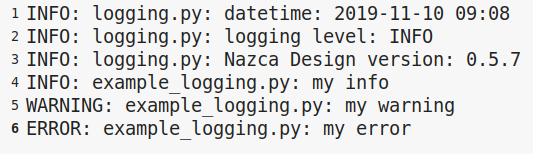In this example we show how to log your layout.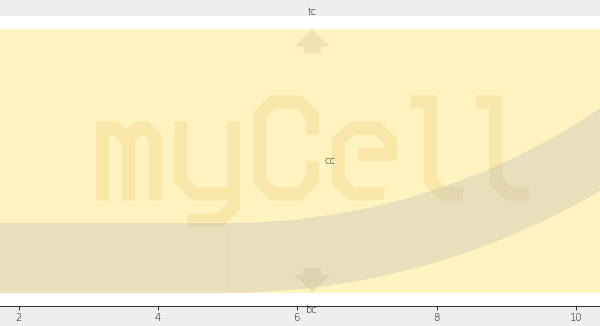In this example we show how to create xsections and layers